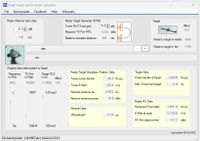With this calculator you can calculate RF parameters in a radar range depending on:

• Frequency
• Target range
• Target RCS
• Antenna gain
• TX Power
With this calculator you can calculate and verify RF levels on a Radar Target Simulation range. Let us first define what a Radar Simulation range is.

The simulator (an electronic device creating virtual targets on a Radar under test) is located in the middle (between the Radar and the Virtual Target) where you see the two coupled antennas. This is the equivalent of a radar target simulator receiving the transmitted pulse from the radar (left) which after signal processing (Adding delay, speed and Doppler shift) re-transmits the pulse back to the Radar so that by electronic means a flying target (on the right) is detected by the Radar.

Based on the target simulation ( Radar Cross Section, distance and target speed) the equivalent numbers are calculated for the simulation position for antenna gain, required transmit power all depending on the distance between the Radar and the target simulation position.

The Radar parameters, Frequency, Power, Antenna gain can be (pre)set for various common Radar frequency bands.>100 RF Calculators
WinRFCalc, the best RF calculator toolbox for Windows